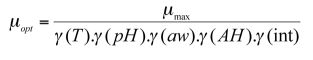# Growth models fitting

## Growth models fitting

Modelling bacterial growth can be split into several levels.

The first level is called the primary growth model which describes growth as a function of time. The main parameters of these models are the growth rate (µmax) and lag time (lag).

The secondary growth model describes the influence of various environmental factors over growth (µmax et lag) that are caused by environmental factors. It takes into account temperature, pH, aw and some growth inhibitors, such as organic acids, which are the main factors having major effects on bacterial behaviour. All other minor factors are incorporated in the food matrix effect.

Primary growth models

 The growth kinetics of a bacterial population are traditionally divided into three phases : lag phase exponential growth phase stationary phase The change in population size over time is described by the primary model. Rosso (1996) demonstrated that the logistic model using delay and rupture was a model that, compared to those available at the time, provided a very good accuracy using only 4 descriptive parameters: lag time (lag), growth rate (µmax), initial population size (N0) and the maximum population size (Nmax). This model is used by Sym’Previus.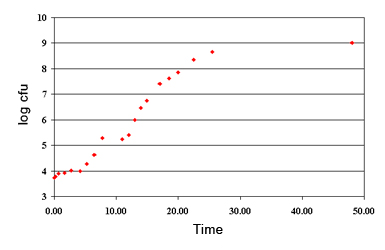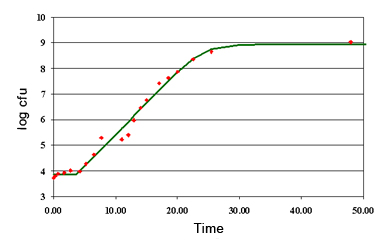Secondary growth models

 Environmental factors The secondary models describe the changes of environmental factors to bacterial behaviour over time. Secondary growth models: cardinal models Rosso et al. (1993) introduced a model that described the influence of environmental factors on the growth rate using only parameters that were biologically significant. This model then became widely known as the cardinal model (Rosso, 1998) and allowed the effect of 3 factors to be described: temperature, pH and aw.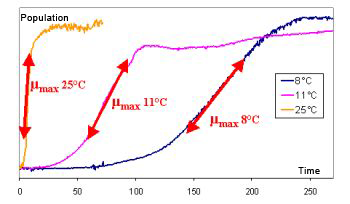Gamma temperature cardinal model (Rosso et al., 1993) :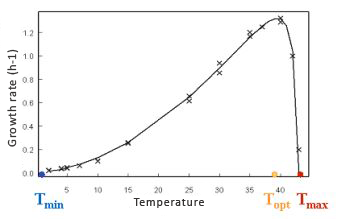T : Temperature

Tmin : Minimal growth temperature

Topt : Optimal growth temperature

Tmax : Maximal growth temperature

Secondary growth models: inhibitor modelOrganic acids affect bacterial growth in two different ways: by changing pH and by a specific inhibition.

The first effect is taken into account by the pH part of the cardinal model described above.
The second is the inhibitor effect of the non-dissociated form of the acid. Le Marc et al. (2002) and Coroller et al. (2005) suggested a model containing only two parameters.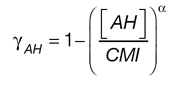[AH] : Concentration en acide organique non-dissocié
CMI : Concentration Minimale Inhibitrice d’acide non dissocié
alpha : Paramètre de forme

Interactions between environmental factorsThe interactions between environmental factors are described as an independent module. Proposed by Le Marc et al. (2001) then modified by Augustin et al. (2005), this function does not require any additional parameter.
This interaction module uses only the micro-organism’s physiological parameters that have already been used by the previous models.

Food matrix effect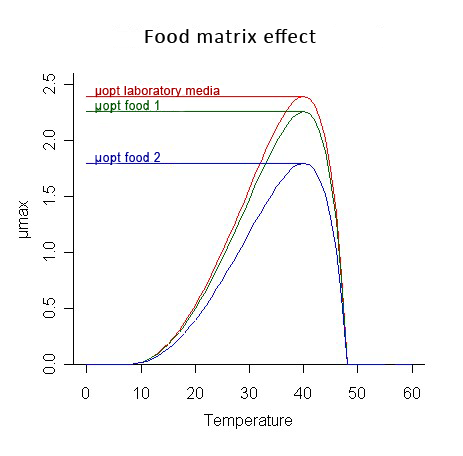All the factors that are not taken into account in the models are incorporated into the “food matrix”. The µopt parameter characterises this matrix effect. It is the growth rate that is observed in ideal conditions for the micro-organism.

It is calculated from a growth kinetic for the specific foodstuff, e.g. during a growth test.

It is possible to simulate the growth under any other formulation of storage conditions using the µopt.